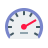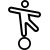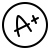Anop Hindi Typing Tutor: Contact Us here you can report about your query or any kind of problem facing in Operating or using the Software. Users can also report the bugs related to Anop Hindi Typing Tutor in this section

Exercise Name {{this.exeDataAll.test_name}}
{{exeLetter}}Click to {{ this.status == 'paused' ? 'Resume' : 'Start'}}
{{ Math.round(this.f_speed)}}WPMNet Speed Gross Speed {{ Math.round(this.f_g_speed)}}WPM## Formula Used:Gross Speed = (Total Character Typed / 5) / Time TakenNet Speed = Gross Speed - [(Wrong Character Typed / 5) / Time Taken]Backspace, Space, Shift Keypress, Skipped Words are not counted for calculation.

# ANOP TYPING TUTOR{{ this.exerciseData.length }} Total Words
{{ this.c_Word + 1 }} Current Word
{{ this.f_g_speed>0 ? Math.round(this.f_g_speed) : '0' }} Gross Speed WPM
{{ Math.round(this.f_speed) }} Net Speed WPM
{{ Math.round(this.accuracy) }} Accuracy %
{{ this.time }}
{{ this.no_r_Word }} Words Right
{{ this.no_w_Word }} Words Wrong
{{ this.no_s_Word }} Words Skip
{{ this.no_r_Letter }} Letters Right
{{ this.no_w_Letter }} Letters Wrong
Tutorial Name {{this.dataProp.tutorial_name}}Click to {{ !this.running && this.status == 'running' ? 'Resume' : 'Start'}}
{{ Math.round(this.f_speed)}}WPMNet Speed Gross Speed {{ Math.round(this.f_g_speed)}}WPM## Formula Used:Gross Speed = (Total Character Typed / 5) / Time TakenNet Speed = Gross Speed - [(Wrong Character Typed / 5) / Time Taken]Backspace, Space, Shift Keypress, Skipped Words are not counted for calculation.
{{systemWord}} {{userWord}}
{{ this.var_lf_1 ? this.upCommingKey : "^" }} {{ this.var_lf_2 ? this.upCommingKey : "^" }} {{ this.var_lf_3 ? this.upCommingKey : "^" }} {{ this.var_lf_4 ? this.upCommingKey : "^" }} {{ this.var_rf_1 ? this.upCommingKey : "^" }} {{ this.var_rf_2 ? this.upCommingKey : "^" }} {{ this.var_rf_3 ? this.upCommingKey: "^" }} {{ this.var_rf_4 ? this.upCommingKey : "^" }}~
`
! !
1
@ /
2
# रु
3
\$ +
4
% :
5
^
6
& -
7
*
8
( ;
9
) द्ध
0
_ ऋ़
. -
+
त्र =
Backspace
Tab
Q
q
W
w
E म्
e
R त्
r
T ज्
t
Y ल्
y
U न्
u
I प्
i
O व्
o
P च्
p
{ क्ष्
ख् [
} द्व
, ]
| द्य
? \
Caps
Lock
A
a
S
s
D क्
d
F थ्
f ि
G
g
H भ्
h
J श्र
j
K ज्ञ
k
L स्
l
: रू
;
" ष्
श् '
Enter
Shift
Z Z
z z
X ग्
x
C ब्
c
V
v
B
b
N
n
M
m
<
,
>
ण् .
? घ्
ध् /
Shift
Ctrl
Win
Alt
Space
Alt
Win
Ctrl

# ANOP TYPING TUTOR{{ this.tuteData.length }} Total Words
{{ this.tuteCursur + 1 }} Current Word
{{ this.f_g_speed>0 ? Math.round(this.f_g_speed) : '0' }} Gross Speed WPM
{{ Math.round(this.f_speed) }} Net Speed WPM
{{ Math.round(this.accuracy) }} Accuracy %
{{ this.time }}
{{ this.no_c_right }} Letters Right
{{ this.no_c_wrong }} Letters Wrong